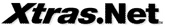The .Net Components, Tools, and Rewards Microsoft .Net Developers Deserve

# Mono Calculator

### Ideal for web pages where any type of numeric input is required

From Mono Ltd

#### Write a Review?

Use the Calculator control to allow a user of a web forms page to process simple arithmetic operations where any type of numeric input is required. Mono Calculator control mimics the Standard Windows Calculator by allowing a user to add, subtract, multiply and divide numbers. Additional operations include: computing the square root of a number, inverse of a number, calculating percentage, etc. Memory related buttons store (clear, recall, add) the current value in memory.

The Display property determines how the control appears to the user. The Movable property determines whether the Calculator can be moved (onyl when Display equals Display.PopUp)